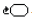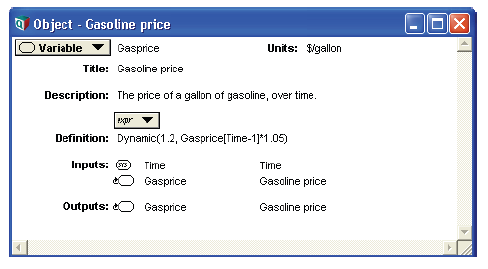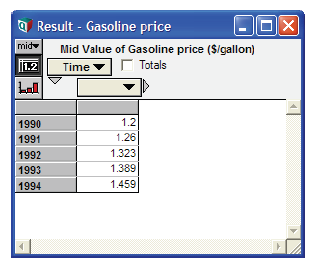# Dynamic function

## Dynamic(initial1, initial2..., initialn, expr)

Performs dynamic simulation, calculating the value of its defined variable at each element of Time. The result of Dynamic() is an array, indexed by Time.

«Initial1», ...«initialn» are the values of the variable for the first n time periods. «expr» is an expression giving the value of the variable for each subsequent time period. «expr» can refer to the variable in earlier time periods, that is, contain its own identifier in its definition. If variable Var is defined using Dynamic, «expr» can be a function of Var[Time - k] or Self[Time - k], where k is an expression that evaluates to an integer between 1 and t, and t is the time step at which «expr» is being evaluated.

Tip

Square brackets ([ ]) are necessary around Time - t.

The Dynamic function must appear at the topmost level of a definition. It cannot be used inside another expression.

When a dynamic variable refers to itself, it appears in its own list of inputs and outputs, with a symbol for cyclic dependency:.

Library: Special

When to use: Use Dynamic() for defining variables that are cyclically dependent. There are only two functions in Analytica that allow a cycle to be formed, in which a variable can refer to its own value or to other variables that depend on it. Those two functions are Dynamic() and Iterate(). Only dynamic variables can compute their value based on the values at at earlier time periods.

Example: Dynamic() can be used to calculate the effect of inflation on the price of gasoline in the years 1990 to 1994.

If the initial value is \$1.20 per gallon and the rate of inflation is 5% per year, then Gasprice can be defined as: Dynamic(1.2, Gasprice[Time - 1]*1.05) or Dynamic(1.2, Self[Time - 1]*1.05).Clicking the Result button and viewing the mid value as a table displays the following results.For 1990, Analytica uses the initial value of Gasprice (1.2). For each subsequent year, Analytica multiplies the value of Gasprice at [Time - 1] by 1.05 (the 5 percent inflation rate).

## x[Time - k]

Given a variable x and brackets enclosing Time minus an integer «k», returns the value for «x», «k» time periods back from the current time period. This function is only valid for variables defined using the Dynamic function.

Library: Special